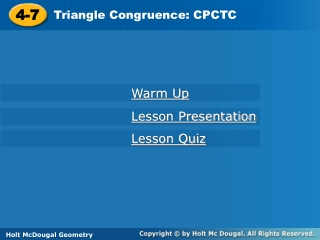DownloadDownload Presentation4-7

# 4-7

Download Presentation## 4-7

- - - - - - - - - - - - - - - - - - - - - - - - - - - E N D - - - - - - - - - - - - - - - - - - - - - - - - - - -
##### Presentation Transcript

1. 4-7 Triangle Congruence: CPCTC Holt Geometry Warm Up Lesson Presentation Lesson Quiz Holt McDougal Geometry

2. EF 17 Warm Up 1. If ∆ABC  ∆DEF, then A  ? and BC  ? . 2. What is the distance between (3, 4) and (–1, 5)? 3. If 1  2, why is a||b? 4.List methods used to prove two triangles congruent. D Converse of Alternate Interior Angles Theorem SSS, SAS, ASA, AAS, HL

3. Objective Use CPCTC to prove parts of triangles are congruent.

4. Vocabulary CPCTC

5. CPCTCis an abbreviation for the phrase “Corresponding Parts of Congruent Triangles are Congruent.” It can be used as a justification in a proof after you have proven two triangles congruent.

6. Remember! SSS, SAS, ASA, AAS, and HL use corresponding parts to prove triangles congruent. CPCTC uses congruent triangles to prove corresponding parts congruent.

7. Therefore the two triangles are congruent by SAS. By CPCTC, the third side pair is congruent, so AB = 18 mi. Example 1: Engineering Application A and B are on the edges of a ravine. What is AB? One angle pair is congruent, because they are vertical angles. Two pairs of sides are congruent, because their lengths are equal.

8. Check It Out! Example 1 A landscape architect sets up the triangles shown in the figure to find the distance JK across a pond. What is JK? One angle pair is congruent, because they are vertical angles. Two pairs of sides are congruent, because their lengths are equal.Therefore the two triangles are congruent by SAS. By CPCTC, the third side pair is congruent, so JK = 41 ft.

9. Given:YW bisects XZ, XY YZ. Z Example 2: Proving Corresponding Parts Congruent Prove:XYW  ZYW

10. ZW WY Example 2 Continued

11. Given:PR bisects QPS and QRS. Prove:PQ  PS Check It Out! Example 2

12. QRP SRP QPR  SPR PR bisects QPS and QRS RP PR Reflex. Prop. of  Def. of  bisector Given ∆PQR  ∆PSR ASA PQPS CPCTC Check It Out! Example 2 Continued

13. Helpful Hint Work backward when planning a proof. To show that ED || GF, look for a pair of angles that are congruent. Then look for triangles that contain these angles.

14. Given:NO || MP, N P Prove:MN || OP Example 3: Using CPCTC in a Proof

15. 1. N  P; NO || MP 3.MO  MO 6.MN || OP Example 3 Continued Statements Reasons 1. Given 2. NOM  PMO 2. Alt. Int. s Thm. 3. Reflex. Prop. of  4. ∆MNO  ∆OPM 4. AAS 5. NMO  POM 5. CPCTC 6. Conv. Of Alt. Int. s Thm.

16. Given:J is the midpoint of KM and NL. Prove:KL || MN Check It Out! Example 3

17. 1.J is the midpoint of KM and NL. 2.KJ  MJ, NJ  LJ 6.KL || MN Check It Out! Example 3 Continued Statements Reasons 1. Given 2. Def. of mdpt. 3. KJL  MJN 3. Vert. s Thm. 4. ∆KJL  ∆MJN 4. SAS Steps 2, 3 5. LKJ  NMJ 5. CPCTC 6. Conv. Of Alt. Int. s Thm.

18. Example 4: Using CPCTC In the Coordinate Plane Given:D(–5, –5), E(–3, –1), F(–2, –3), G(–2, 1), H(0, 5), and I(1, 3) Prove:DEF  GHI Step 1 Plot the points on a coordinate plane.

19. Step 2 Use the Distance Formula to find the lengths of the sides of each triangle.

20. So DEGH, EFHI, and DFGI. Therefore ∆DEF  ∆GHI by SSS, and DEF  GHI by CPCTC.

21. Check It Out! Example 4 Given:J(–1, –2), K(2, –1), L(–2, 0), R(2, 3), S(5, 2), T(1, 1) Prove: JKL RST Step 1 Plot the points on a coordinate plane.

22. RT = JL = √5, RS = JK = √10, and ST = KL = √17. So ∆JKL ∆RST by SSS. JKL RST by CPCTC. Check It Out! Example 4 Step 2 Use the Distance Formula to find the lengths of the sides of each triangle.

23. Lesson Quiz: Part I 1.Given: Isosceles ∆PQR, base QR, PAPB Prove:AR BQ

24. Statements Reasons 1. Isosc. ∆PQR, base QR 1. Given 2.PQ = PR 2. Def. of Isosc. ∆ 3.PA = PB 3. Given 4.P  P 4. Reflex. Prop. of  5.∆QPB  ∆RPA 5. SAS Steps 2, 4, 3 6.AR = BQ 6. CPCTC Lesson Quiz: Part I Continued

25. Lesson Quiz: Part II 2. Given: X is the midpoint of AC . 1 2 Prove: X is the midpoint of BD.

26. Statements Reasons 1.X is mdpt. of AC. 1  2 1. Given 2.AX = CX 2. Def. of mdpt. 3.AX  CX 3. Def of  4. AXD  CXB 4. Vert. s Thm. 5.∆AXD  ∆CXB 5. ASA Steps 1, 4, 5 6.DX  BX 6. CPCTC 7. Def. of  7.DX = BX 8.X is mdpt. of BD. 8. Def. of mdpt. Lesson Quiz: Part II Continued

27. DE = GH = √13, DF = GJ = √13, EF = HJ = 4, and ∆DEF ∆GHJ by SSS. Lesson Quiz: Part III 3. Use the given set of points to prove ∆DEF  ∆GHJ: D(–4, 4), E(–2, 1), F(–6, 1), G(3, 1), H(5, –2), J(1, –2).